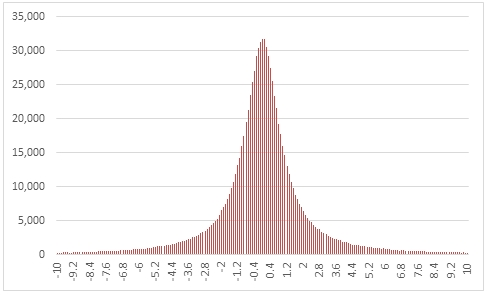# SQL Server random Cauchy distribution

RANDCAUCHY

Updated: 31 March 2014

Use the table-valued function RANDCAUCHY to generate a sequence of random numbers from a Cauchy distribution with locations @mu and scale @sig.
Syntax
SELECT * FROM [wctMath].[wct].[RANDCAUCHY](
<@Rows, int,>
,<@mu, float,>
,<@sig, float,>)
Arguments
@Rows
the number of rows to generate. @Rows must be of the type int or of a type that implicitly converts to int.
@mu
the location parameter. @mu must be of the type float or of a type that implicitly converts to float.
@sig
the scale parameter. @sig must be of the type float or of a type that implicitly converts to float.
Return Types
RETURNS TABLE (
[Seq] [int] NULL,
[X] [float] NULL
)
Remarks
·         @sig must be greater than zero.
·         If @mu is NULL then @mu is set to 0.
·         If @sig is NULL then @sig is set to 1.
·         If @Rows is less than 1 then no rows are returned.
Examples
In this example we create a sequence 1,000,000 random numbers rounded to one decimal place from a Cauchy distribution with @mu = 0 and @sig = 1, COUNT the results, paste them into Excel where the values are between -10 and 10 and graph them.
SELECT
X,
COUNT(*) as COUNT
FROM (
SELECT
ROUND(X,1) as X
FROM wct.RANDCAUCHY(
1000000, --@Rows
0,       --@mu
1,       --@sig
)
)n
WHERE
X BETWEEN -10 AND 10
GROUP BY
X
ORDER BY
1

This produces the following result.See Also

### PricingCopyright 2008-2021 Westclintech LLC         Privacy Policy        Terms of Service